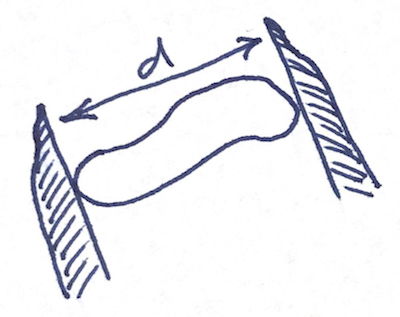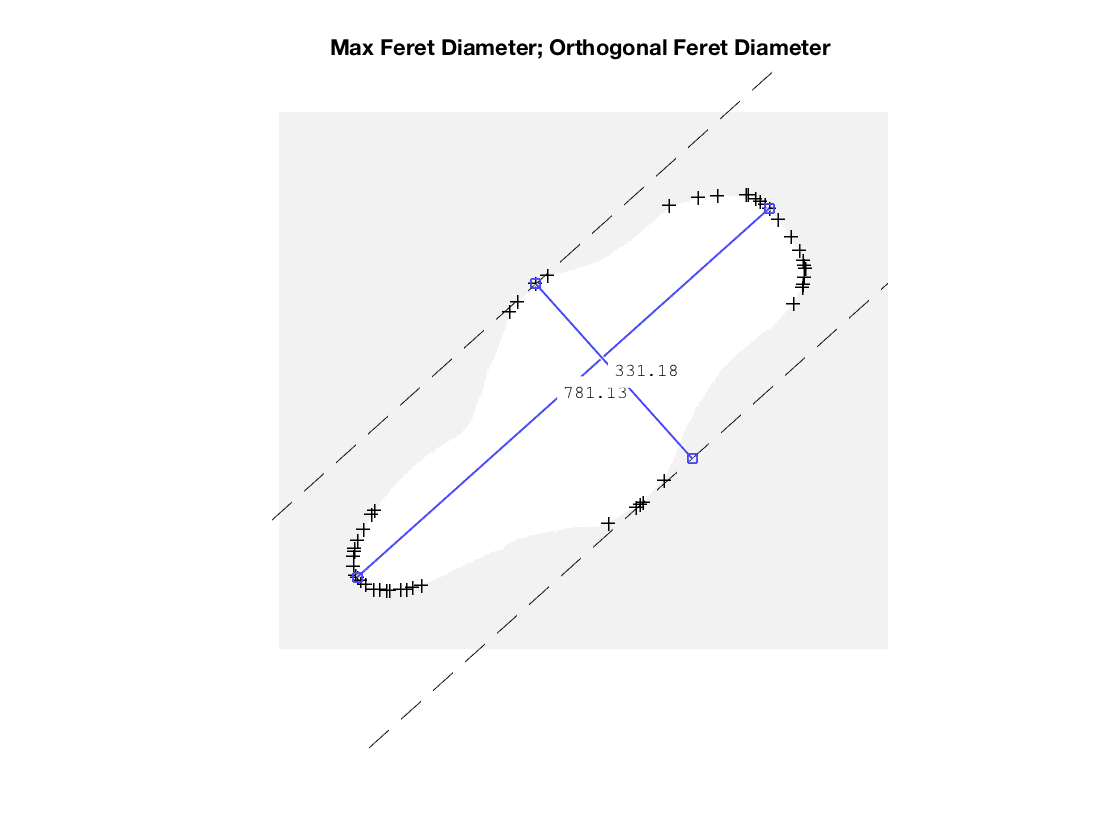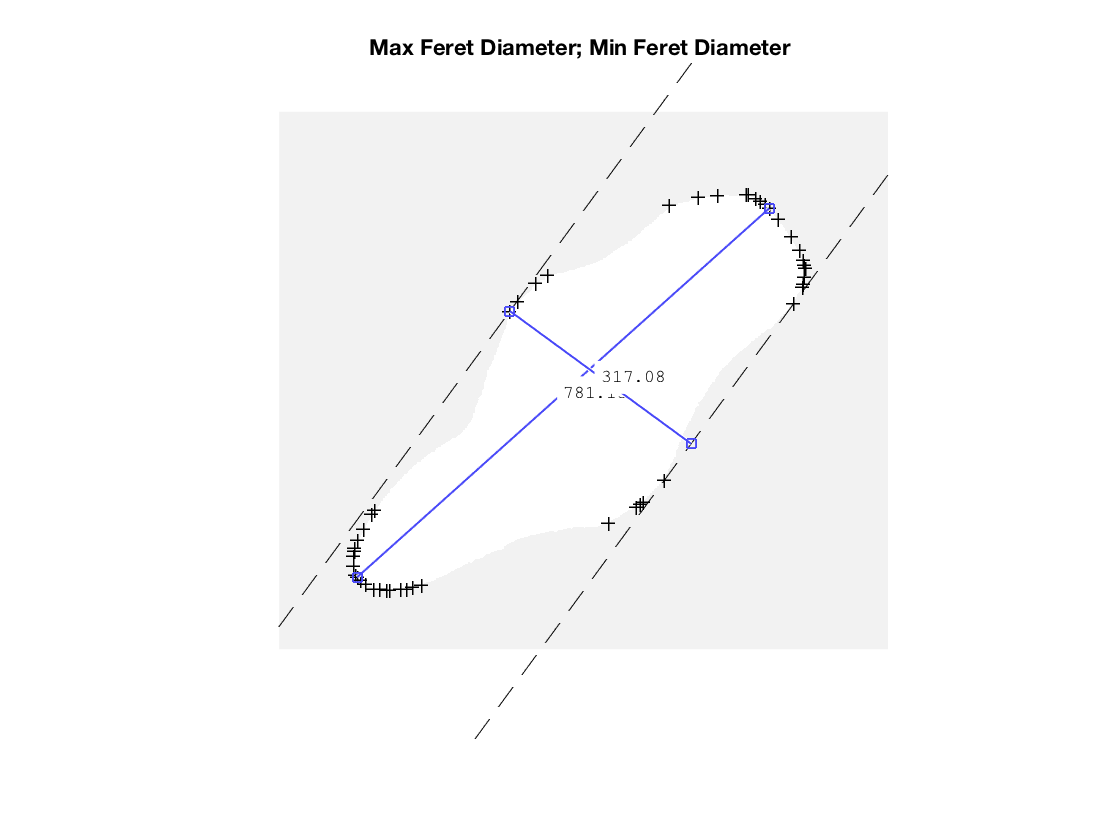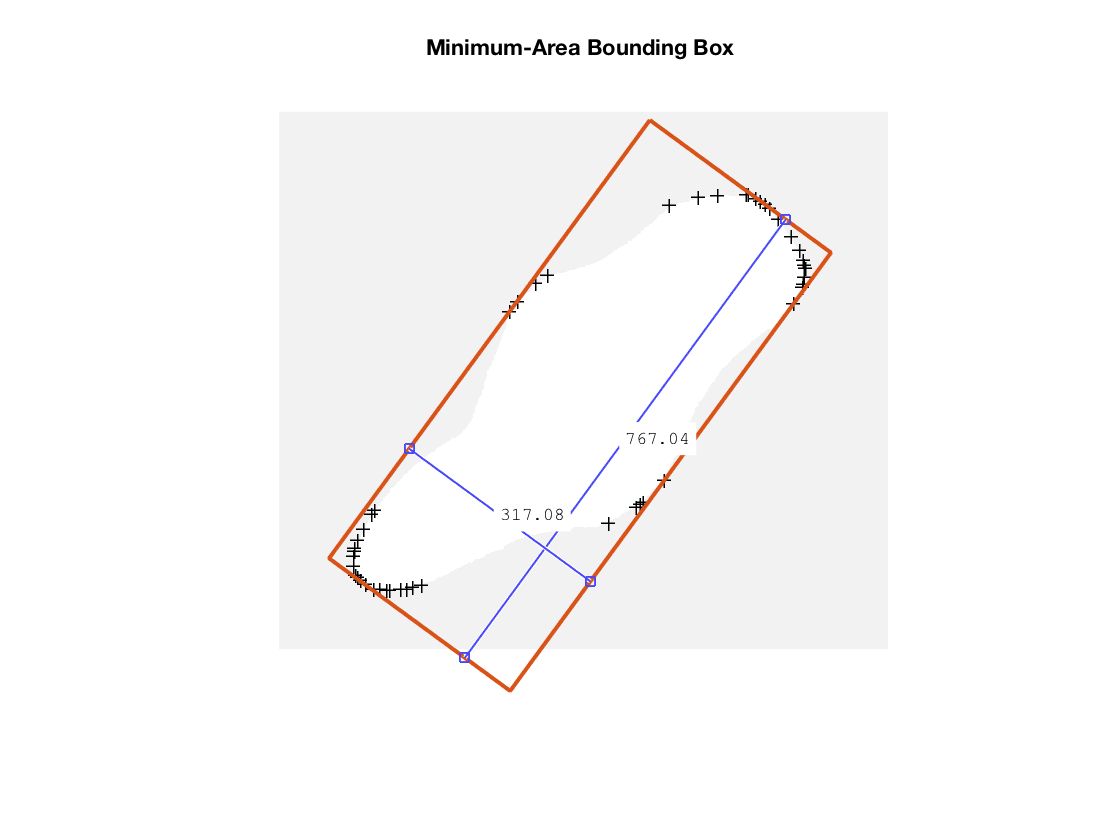# Feret Properties – Wrapping Up

Today I want to finish up my long-running discussion of Feret diameters. (Previous posts: 29-Sep-2017, 24-Oct-2017, 20-Feb-2018, and 16-Mar-2018.)

Recall that the Feret diameter measures the extent of an object along a particular direction. The diagram below illustrates the concept. Place the object to be measured inside the jaws of a caliper, with the caliper oriented at a specified angle. Close the jaws tightly on the object while maintaining that angle. The distance between the jaws is the Feret diameter at that angle.The maximum Feret diameter and minimum Feret diameter measure the maximum and minimum width of an object. In previous posts, I talked about how to identify antipodal vertex pairs from the set the convex hull vertices to speed up the process of finding these maximum and minimum measures. I also considered the various assumptions one can make about the shape of an individual pixel and how those assumptions can affect the results. (In the rest of this post, I'll assume a diamond pixel shape, as suggested by Cris.)

Let's look at these several measurements for a particular object.

bw = imread('shape.png');
imshow(bw)visualizeFeretProperties(bw)The first diagram above shows the maximum Feret diameter and its orientation. It also shows the Feret diameter at the angle that is orthogonal to the maximum diameter.

The second diagram is similar, except that it shows the minimum Feret diameter instead of the orthogonal diameter. You can see that they are not the same, and, in general, they won't be.

The third diagram shows the minimum-area bounding box, which can be found using a search procedure similar to the minimum Feret diameter. In this diagram, notice that the bounding box is not exactly aligned with the direction of the maximum Feret dimension. In general, they won't necessarily be aligned, and then the length of the minimum-area bounding box will be less than the maximum Feret dimension.

Below is a function I wrote that adds Feret diameter properties to the table returned by regionprops (using the 'table' option). It makes use of the algorithmic functions that you can find in my previous posts.

T = regionprops('table',bw,'PixelList')

T=1×1 table
PixelList
_________________

[163875×2 double]


T = feretProperties(T)

T=1×12 table
PixelList        MaxFeretDiameter    MaxFeretDiameterEndpoints    MaxFeretDiameterOrientation    MinFeretDiameter    MinFeretDiameterTrianglePoints    MinFeretDiameterOrientation    OrthogonalDiameter    OrthogonalDiameterLowerPoints    OrthogonalDiameterUpperPoints    MinAreaBoundingBox    MinAreaBoundingBoxArea
_________________    ________________    _________________________    ___________________________    ________________    ______________________________    ___________________________    __________________    _____________________________    _____________________________    __________________    ______________________

[163875×2 double]         781.13               [2×2 double]                     -41.835                   317.08                  [3×2 double]                       -143.8                     331.18                  [1×2 double]                     [1×2 double]                [5×2 double]             2.4321e+05



I hope that at least a few of you enjoyed this diversion into algorithms associated with object shape measurement.

### Appendix: Functions Used

function T = feretProperties(T)
% Copyright 2017-2018 The MathWorks, Inc.

maxfd = zeros(height(T),1);
maxfd_endpoints = cell(height(T),1);
maxfd_orientation = zeros(height(T),1);

minfd = zeros(height(T),1);
minfd_triangle_points = cell(height(T),1);
minfd_orientation = zeros(height(T),1);

minod = zeros(height(T),1);
minod_lower_points = cell(height(T),1);
minod_upper_points = cell(height(T),1);

minbb = cell(height(T),1);
minbb_a = zeros(height(T),1);

for k = 1:height(T)
pixels = T.PixelList{k};
V = pixelHull(pixels,'diamond');
pairs = antipodalPairs(V);
[maxfd(k),maxfd_endpoints{k}] = maxFeretDiameter(V,pairs);
points = maxfd_endpoints{k};
e = points(2,:) - points(1,:);
maxfd_orientation(k) = atan2d(e(2),e(1));

[minfd(k),minfd_triangle_points{k}] = minFeretDiameter(V,pairs);
points = minfd_triangle_points{k};
e = points(2,:) - points(1,:);
minfd_orientation(k) = mod(thetad + 180 + 90,360) - 180;

[minod(k),minod_lower_points{k},minod_upper_points{k}] = ...
feretDiameter(V,maxfd_orientation(k)+90);

[minbb{k},minbb_a(k)] = minAreaBoundingBox(V,pairs);
end

T.MaxFeretDiameter = maxfd;
T.MaxFeretDiameterEndpoints = maxfd_endpoints;
T.MaxFeretDiameterOrientation = maxfd_orientation;
T.MinFeretDiameter = minfd;
T.MinFeretDiameterTrianglePoints = minfd_triangle_points;
T.MinFeretDiameterOrientation = minfd_orientation;
T.OrthogonalDiameter = minod;
T.OrthogonalDiameterLowerPoints = minod_lower_points;
T.OrthogonalDiameterUpperPoints = minod_upper_points;
T.MinAreaBoundingBox = minbb;
T.MinAreaBoundingBoxArea = minbb_a;
end

function [bb,A] = minAreaBoundingBox(V,antipodal_pairs)
% Copyright 2017-2018 The MathWorks, Inc.

if nargin < 2
antipodal_pairs = antipodalPairs(V);
end

n = size(antipodal_pairs,1);
p = antipodal_pairs(:,1);
q = antipodal_pairs(:,2);

A = Inf;

for k = 1:n
if k == n
k1 = 1;
else
k1 = k+1;
end

pt1 = [];
pt2 = [];
pt3 = [];

if (p(k) ~= p(k1)) && (q(k) == q(k1))
pt1 = V(p(k),:);
pt2 = V(p(k1),:);
pt3 = V(q(k),:);

elseif (p(k) == p(k1)) && (q(k) ~= q(k1))
pt1 = V(q(k),:);
pt2 = V(q(k1),:);
pt3 = V(p(k),:);
end

if ~isempty(pt1)
% Points pt1, pt2, and pt3 are possibly on the minimum-area
% bounding box, with points pt1 and pt2 forming an edge coincident with
% the bounding box. Call the height of the triangle with base
% pt1-pt2 the height of the bounding box, h.

h = triangleHeight(pt1,pt2,pt3);

pt1pt2_direction = atan2d(pt2(2)-pt1(2),pt2(1)-pt1(1));

w = feretDiameter(V,pt1pt2_direction);

A_k = h*w;
if (A_k < A)
A = A_k;
end
end
end

% Rotate all the points so that pt1-pt2 for the minimum bounding box points
% straight up.

cr = cosd(r);
sr = sind(r);
R = [cr -sr; sr cr];

Vr = V * R';

xr = Vr(:,1);
yr = Vr(:,2);
xmin = min(xr);
xmax = max(xr);
ymin = min(yr);
ymax = max(yr);

bb = [ ...
xmin ymin
xmax ymin
xmax ymax
xmin ymax
xmin ymin ];

% Rotate the bounding box points back.
bb = bb * R;
end

function h = triangleHeight(P1,P2,P3)
% Copyright 2017-2018 The MathWorks, Inc.

h = 2 * abs(signedTriangleArea(P1,P2,P3)) / norm(P1 - P2);
end

function area = signedTriangleArea(A,B,C)
% Copyright 2017-2018 The MathWorks, Inc.

area = ( (B(1) - A(1)) * (C(2) - A(2)) - ...
(B(2) - A(2)) * (C(1) - A(1)) ) / 2;
end

function [d,V1,V2] = feretDiameter(V,theta)
% Copyright 2017-2018 The MathWorks, Inc.

% Rotate points so that the direction of interest is vertical.

alpha = 90 - theta;

ca = cosd(alpha);
sa = sind(alpha);
R = [ca -sa; sa ca];

% Vr = (R * V')';
Vr = V * R';

y = Vr(:,2);
ymin = min(y,[],1);
ymax = max(y,[],1);

d = ymax - ymin;

if nargout > 1
V1 = V(y == ymin,:);
V2 = V(y == ymax,:);
end

function [d,end_points] = maxFeretDiameter(P,antipodal_pairs)
% Copyright 2017-2018 The MathWorks, Inc.

if nargin < 2
antipodal_pairs = antipodalPairs(P);
end

v = P(antipodal_pairs(:,1),:) - P(antipodal_pairs(:,2),:);
D = hypot(v(:,1),v(:,2));
[d,idx] = max(D,[],1);
P1 = P(antipodal_pairs(idx,1),:);
P2 = P(antipodal_pairs(idx,2),:);

end_points = [P1 ; P2];
end

function [d,triangle_points] = minFeretDiameter(V,antipodal_pairs)
% Copyright 2017-2018 The MathWorks, Inc.

if nargin < 2
antipodal_pairs = antipodalPairs(V);
end

n = size(antipodal_pairs,1);
p = antipodal_pairs(:,1);
q = antipodal_pairs(:,2);

d = Inf;
triangle_points = [];

for k = 1:n
if k == n
k1 = 1;
else
k1 = k+1;
end

pt1 = [];
pt2 = [];
pt3 = [];

if (p(k) ~= p(k1)) && (q(k) == q(k1))
pt1 = V(p(k),:);
pt2 = V(p(k1),:);
pt3 = V(q(k),:);

elseif (p(k) == p(k1)) && (q(k) ~= q(k1))
pt1 = V(q(k),:);
pt2 = V(q(k1),:);
pt3 = V(p(k),:);
end

if ~isempty(pt1)
% Points pt1, pt2, and pt3 form a possible minimum Feret diameter.
% Points pt1 and pt2 form an edge parallel to caliper direction.
% The Feret diameter orthogonal to the pt1-pt2 edge is the height
% of the triangle with base pt1-pt2.

d_k = triangleHeight(pt1,pt2,pt3);
if d_k < d
d = d_k;
triangle_points = [pt1; pt2; pt3];
end
end
end
end


|

### 댓글

댓글을 남기려면 링크 를 클릭하여 MathWorks 계정에 로그인하거나 계정을 새로 만드십시오.